2020-05-28 10:14:33

## 手把手带你走进卷积神经网络！

30 / 0 / 0 / 0

#### CNN

https://victorzhou.com/blog/intro-to-neural-networks/

### 1动机

CNN的经典用例是执行图像分类，例如查看宠物的图像并确定它是猫还是狗。这是一项看似非常简单的任务，你可能会有这样的疑惑：为什么不使用普通的神经网络呢？不得不说这是一个好问题。

### 2 数据集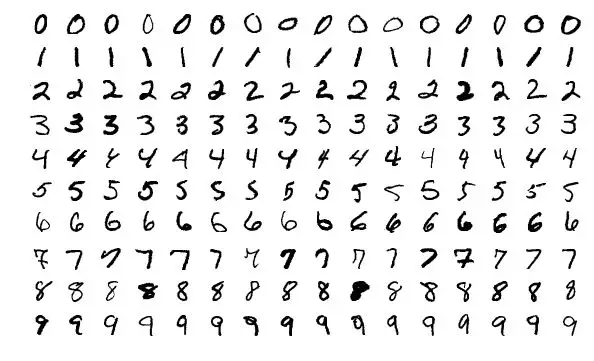<>来自MNIST数据集的样本图像

MNIST数据集中的每个图像都是28x28，其中包含了一个居中的灰度数字。说实话，一个正常的神经网络实际上可以很好地解决这个问题。你可以将每个图像视为28x28=784维矢量，将其输入到784-dim图层，堆叠一些隐藏图层，最后输出10个节点的输出图层，每个数字1个。

### 3卷积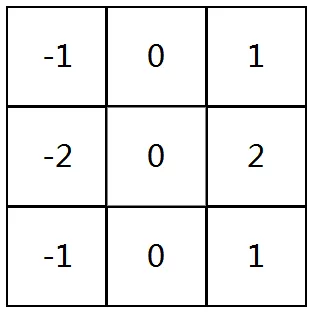<>一个3x3过滤器

• 在某个位置覆盖图像顶部的过滤器；

• 在过滤器中的值与图像中的相应值之间执行逐元素乘法；

• 所有元素（element-wise products）求和，此和是输出图像中目标像素的输出值。

• 重复所有位置。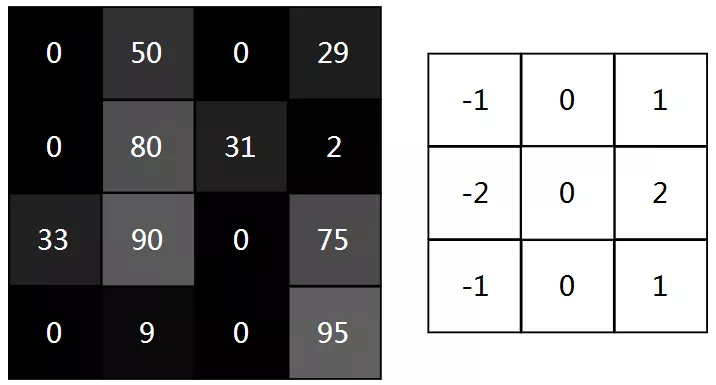<>4x4图像（左）和3x3滤镜（右）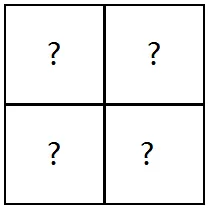<>2x2输出图像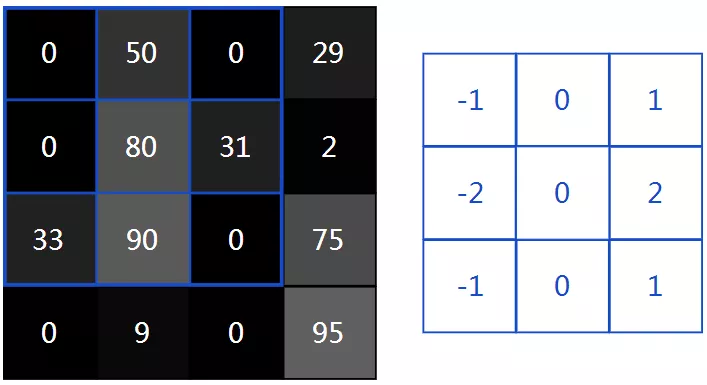<>第1步：将过滤器（右）叠加在图像上方（左）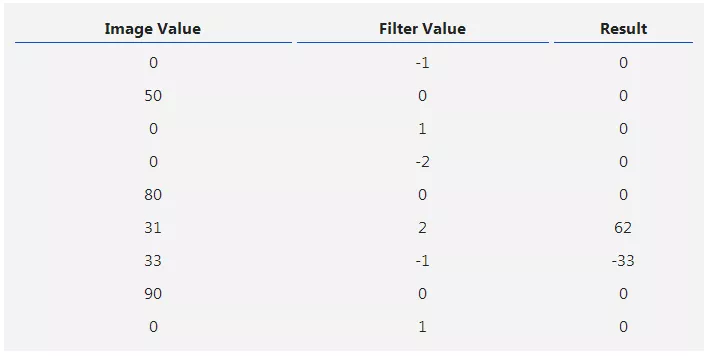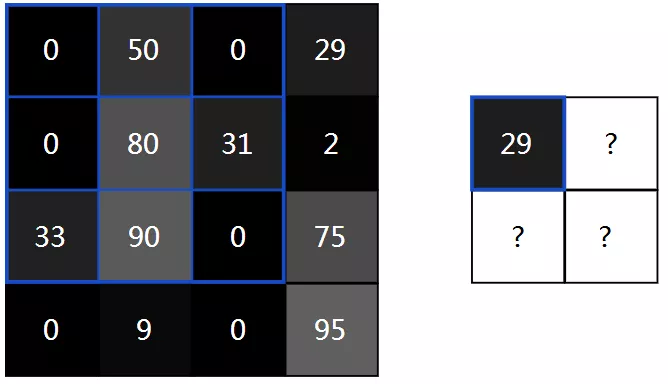3.1这有用吗？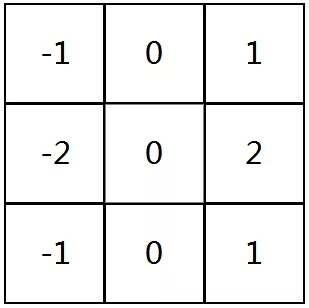<>垂直索贝尔滤波器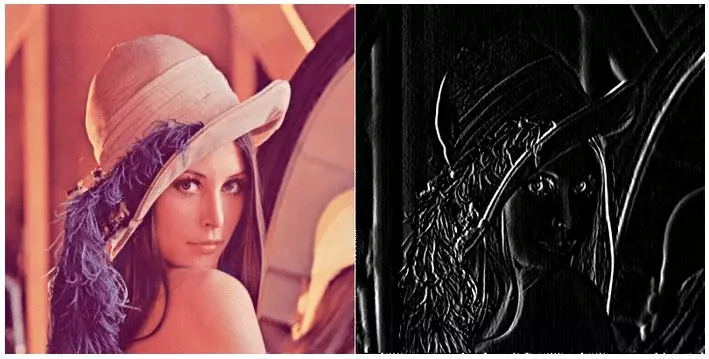<>垂直Sobel滤波器卷积的图像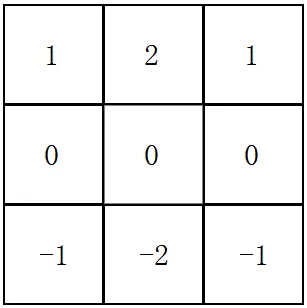<>水平Sobel滤波器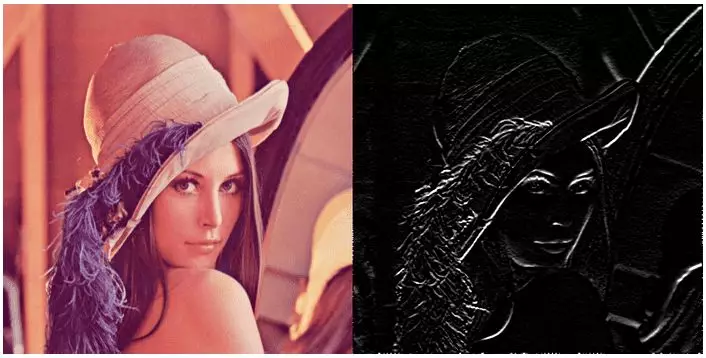<>水平Sobel滤波器卷积的图像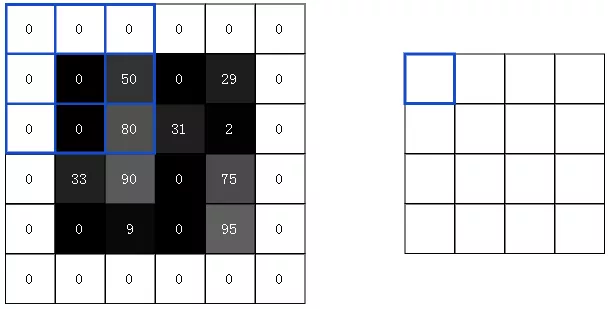<>4x4输入与3x3滤波器卷积，使用填充以产生4x4输出

3.3 Conv图层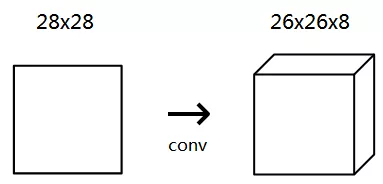conv layer中的4个过滤器中的每一个都会产生26x26输出，因此堆叠在一起它们构成26x26x8的向量。

3.4执行卷积

import numpy as np

class Conv3x3:
# A Convolution layer using 3x3 filters.

def __init__(self, num_filters):
self.num_filters = num_filters

# filters is a 3d array with dimensions (num_filters, 3, 3)
# We divide by 9 to reduce the variance of our initial values
self.filters = np.random.randn(num_filters, 3, 3) / 9  

https://www.quora.com/What-is-an-intuitive-explanation-of-the-Xavier-Initialization-for-Deep-Neural-Networks

class Conv3x3:
# ...

def iterate_regions(self, image):
'''
Generates all possible 3x3 image regions using valid padding.
- image is a 2d numpy array
'''
h, w = image.shape

for i in range(h - 2):
for j in range(w - 2):
im_region = image[i:(i + 3), j:(j + 3)]
yield im_region, i, j

def forward(self, input):
'''
Performs a forward pass of the conv layer using the given input.
Returns a 3d numpy array with dimensions (h, w, num_filters).
- input is a 2d numpy array
'''
h, w = input.shape
output = np.zeros((h - 2, w - 2, self.num_filters))

for im_region, i, j in self.iterate_regions(input):
output[i, j] = np.sum(im_region * self.filters, axis=(1, 2))

return output  

iterate_regions()是一个辅助生成器方法，为我们生成所有有效的3x3图像区域，这对于稍后实现此类的后向部分非常有用。

• im_region：一个包含相关图像区域的3x3阵列。

• self.filters：一个3d数组。

• axis=(1,2)num_filters：我们使用np.sum（）上一步的结果，产生一个长度为1d的数组，其中每个元素包含相应过滤器的卷积结果。

• 我们将结果分配给output[i,j]，其中包含输出中像素的卷积结果（i，j）。

import mnist
from conv import Conv3x3

# The mnist package handles the MNIST dataset for us!
# Learn more at https://github.com/datapythonista/mnist
train_images = mnist.train_images()
train_labels = mnist.train_labels()

conv = Conv3x3(8)
output = conv.forward(train_images)
print(output.shape) # (26, 26, 8)  

#### 4池化（Pooling）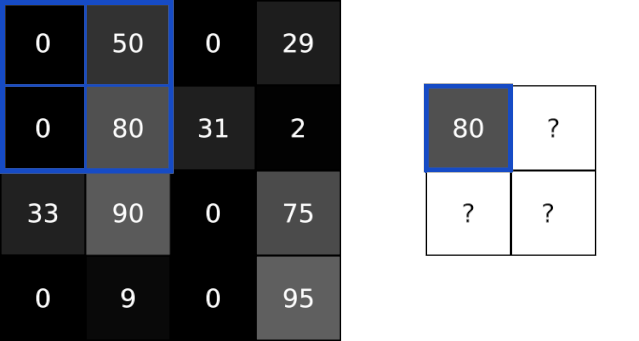<>4x4图像上的最大池（池大小为2）以产生2x2输出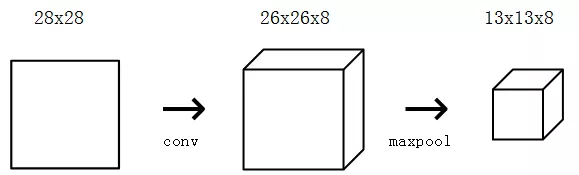4.1执行池化

import numpy as np

class MaxPool2:
# A Max Pooling layer using a pool size of 2.

def iterate_regions(self, image):
'''
Generates non-overlapping 2x2 image regions to pool over.
- image is a 2d numpy array
'''
h, w, _ = image.shape
new_h = h // 2
new_w = w // 2

for i in range(new_h):
for j in range(new_w):
im_region = image[(i * 2):(i * 2 + 2), (j * 2):(j * 2 + 2)]
yield im_region, i, j

def forward(self, input):
'''
Performs a forward pass of the maxpool layer using the given input.
Returns a 3d numpy array with dimensions (h / 2, w / 2, num_filters).
- input is a 3d numpy array with dimensions (h, w, num_filters)
'''
h, w, num_filters = input.shape
output = np.zeros((h // 2, w // 2, num_filters))

for im_region, i, j in self.iterate_regions(input):
output[i, j] = np.amax(im_region, axis=(0, 1))

return output  

import mnist
from conv import Conv3x3
from maxpool import MaxPool2

# The mnist package handles the MNIST dataset for us!
# Learn more at https://github.com/datapythonista/mnist
train_images = mnist.train_images()
train_labels = mnist.train_labels()

conv = Conv3x3(8)
pool = MaxPool2()

output = conv.forward(train_images)
output = pool.forward(output)
print(output.shape) # (13, 13, 8)  

MNIST CNN马上要完成了！

#### 5Softmax

Softmax将任意实际值转换为概率。如果你对其背后的数学有兴趣，自己可以简单了解下，因为它很简单。

5.1用法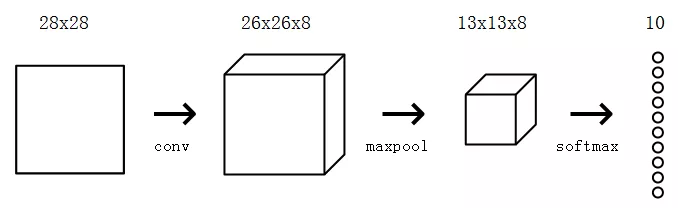5.2交叉熵损失函数

softmax真正做的是帮助我们量化我们对预测的确定程度，这在训练和评估我们的CNN时非常有用。更具体地说，它可以帮我们确定每个预测的正确程度。

5.3实施Softmax

import numpy as np

class Softmax:
# A standard fully-connected layer with softmax activation.

def __init__(self, input_len, nodes):
# We divide by input_len to reduce the variance of our initial values
self.weights = np.random.randn(input_len, nodes) / input_len
self.biases = np.zeros(nodes)

def forward(self, input):
'''
Performs a forward pass of the softmax layer using the given input.
Returns a 1d numpy array containing the respective probability values.
- input can be any array with any dimensions.
'''
input = input.flatten()

input_len, nodes = self.weights.shape

totals = np.dot(input, self.weights) + self.biases
exp = np.exp(totals)
return exp / np.sum(exp, axis=0)  

import mnist
import numpy as np
from conv import Conv3x3
from maxpool import MaxPool2
from softmax import Softmax

# We only use the first 1k testing examples (out of 10k total)
# in the interest of time. Feel free to change this if you want.
test_images = mnist.test_images()[:1000]
test_labels = mnist.test_labels()[:1000]

conv = Conv3x3(8)                  # 28x28x1 -> 26x26x8
pool = MaxPool2()                  # 26x26x8 -> 13x13x8
softmax = Softmax(13 * 13 * 8, 10) # 13x13x8 -> 10

def forward(image, label):
'''
Completes a forward pass of the CNN and calculates the accuracy and
cross-entropy loss.
- image is a 2d numpy array
- label is a digit
'''
# We transform the image from [0, 255] to [-0.5, 0.5] to make it easier
# to work with. This is standard practice.
out = conv.forward((image / 255) - 0.5)
out = pool.forward(out)
out = softmax.forward(out)

# Calculate cross-entropy loss and accuracy. np.log() is the natural log.
loss = -np.log(out[label])
acc = 1 if np.argmax(out) == label else 0

return out, loss, acc

print('MNIST CNN initialized!')

loss = 0
num_correct = 0
for i, (im, label) in enumerate(zip(test_images, test_labels)):
# Do a forward pass.
_, l, acc = forward(im, label)
loss += l
num_correct += acc

# Print stats every 100 steps.
if i % 100 == 99:
print(
'[Step %d] Past 100 steps: Average Loss %.3f | Accuracy: %d%%' %
(i + 1, loss / 100, num_correct)
)
loss = 0
num_correct = 0  

MNIST CNN initialized!
[Step 100] Past 100 steps: Average Loss 2.302 | Accuracy: 11%
[Step 200] Past 100 steps: Average Loss 2.302 | Accuracy: 8%
[Step 300] Past 100 steps: Average Loss 2.302 | Accuracy: 3%
[Step 400] Past 100 steps: Average Loss 2.302 | Accuracy: 12%  

#### 总结

• 介绍了为什么CNN可能对某些问题更有用，例如图像分类；

• 介绍了MNIST手写数字数据集；

• 了解了Conv图层，它将过滤器与图像进行卷积，以产生更有用的输出；

• 了解了Pooling图层，它可以帮助修剪除最有用特征之外的内容；

• 实现了Softmax层，因此我们可以使用交叉熵损失函数。

PS: 如本文对您有疑惑，可加QQ：1752338621 进行讨论。

0
0